In light of the Coronavirus crisis, please help make sure nothing stands in the way of social distancing in the VFX industry.

This petition asks the MPAA to act accordingly:

Sign the petition on Change.org

Be safe, everyone.

## [Fuse] Time Machine Modifier Fuse

Dunn
Moderator
Posts: 486
Joined: Mon Aug 04, 2014 4:27 am
Location: Hamburg, Germany
Been thanked: 1 time
Contact:

### Re: [Fuse] Time Machine Modifier Fuse

Cool, Yeah i did install the new version, but didn´t find anything.But nice to know that someone is using it .. Will maybe add some features soon.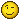Tags:

giorgiokrenkel
Posts: 37
Joined: Sun Sep 27, 2015 2:13 pm
Location: Brasil

### Re: [Fuse] Time Machine Modifier Fuse

Dunn wrote:Cool, Yeah i did install the new version, but didn´t find anything.But nice to know that someone is using it .. Will maybe add some features soon.That would be really nice. More tools for motion graphics!!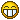SecondMan
Posts: 4229
Joined: Thu Jul 31, 2014 5:31 pm
Been thanked: 58 times
Contact:

## Time Machine 2 is released!

timemachine2.PNG

Well, this has been a while. Time Machine finally gets an update and it's exciting!Version 2 brings Point parameter support. Now you can use Time Machine to retime Point parameters. Using Time Machine's many available modes, motion paths can be constructed in entirely novel new ways.

But more exciting is that as of this version, Time Machine can also be used to retime the actual animated input parameters! As an exciting use case, imagine using Suck Less Audio to modify a parameter. Now you can use Time Machine to retime that motion, effectively looping, reversing, or do any kind of crazy retimes on that sample data itself.

You can switch between the different modes, too. Hover over the buttons to find out what they do.

timemachine2tooltips.PNG

It's the ultimate tool for motion graphics projects and animation (if I may blow my own trumpet a little here)

Time Machine 2 is fully backwards compatible with previous versions with the same core code, so please feel free to update and test.

Time Machine 2 is available through Reactor right now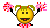You do not have the required permissions to view the files attached to this post.

Dunn
Moderator
Posts: 486
Joined: Mon Aug 04, 2014 4:27 am
Location: Hamburg, Germany
Been thanked: 1 time
Contact:

### Re: [Fuse] Time Machine Modifier Fuse

SecondMan wrote:
Tue Jul 24, 2018 6:43 pm

....As an exciting use case, imagine using Suck Less Audio to modify a parameter. Now you can use Time Machine to retime that motion, effectively looping, reversing, or do any kind of crazy retimes on that sample data itself.

..You just blew my mind !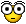Wow !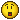good to see this back on track.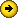A must have for all motion artist out there!
Thanks for the Update SecondMan.SecondMan
Posts: 4229
Joined: Thu Jul 31, 2014 5:31 pm
Been thanked: 58 times
Contact:

## Time Machine 2.1 is here!

While we're at it...Time Machine is a Number Modifier. It's also a Point Modifier. And an Image retimer. And a 3D Scene Retimer. And a 3D Material retimer!

As of today, no more adding TimeStretchers and modifying the Source Time. Time Machine is now also 3 full fledged retiming tools.One click in Reactor and they are all yours! Enjoy!SecondMan
Posts: 4229
Joined: Thu Jul 31, 2014 5:31 pm
Been thanked: 58 times
Contact:

### Re: [Fuse] Time Machine Modifier Fuse

Version 2.11 fixes a potential reliability issue with Resolve 15.

Fusionator
Posts: 1455
Joined: Fri Aug 08, 2014 1:11 pm
Been thanked: 3 times

### Re: [Fuse] Time Machine Modifier Fuse

Did Peter ever give us the means to make a polymorphic fuse? Seems an ideal case. Could add number, mesh, gradient, text, etc. All using the same tool and 99% of the code.

SecondMan
Posts: 4229
Joined: Thu Jul 31, 2014 5:31 pm
Been thanked: 58 times
Contact:

### Re: [Fuse] Time Machine Modifier Fuse

With the DataTypeArray?

Yes, that works now. I've been toying with it a little bit, but I hadn't worked out some stumbling blocks that prevented me to use it with Time Machine. While making this I've had a couple of new ideas so I think I may go back to it.

There won't be a lot less code than there is now, I think, and I believe I'll still have to register it twice, as a Modifier and a Tool. But it would be nice if I could get to one Time Machine tool for Images as well as 3D, that you could connect anything to, on the fly.

So mesh is a datatype too? Available to Fuses?

Fusionator
Posts: 1455
Joined: Fri Aug 08, 2014 1:11 pm
Been thanked: 3 times

### Re: [Fuse] Time Machine Modifier Fuse

Yeah, the main benefit for a user is that they could copy and paste the tool between to inputs of different types. The code reuse would just be in making the input control groups and retiming functions work on any type.

Mesh as in gridwarp mesh.

Dunn
Moderator
Posts: 486
Joined: Mon Aug 04, 2014 4:27 am
Location: Hamburg, Germany
Been thanked: 1 time
Contact:

### Re: [Fuse] Time Machine Modifier Fuse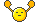Geeez ! 2.1 Rocks !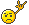grace_mick
Posts: 5
Joined: Sat Aug 10, 2019 4:46 pm
Location: Russia, Moscow
Real name: Dima Dragun
Contact:

### Re: [Fuse] Time Machine Modifier Fuse

Dunn wrote:
Wed Oct 08, 2014 4:25 am
Holy Sh*T!i just started using it .. and i must say .. WOW!!
I have been wanting something like this for a long time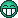What would be cool is to have is some kind of function to overshoot the numbers... creating some kind of bounce effect.
I have a Modifier fuse here that i use for animation.. it´s sort of the basic..basic..basic to what you created ..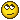AnimationEase.Fuse
It works like... the more Mass + less Bounce = Bounce effect ... & ... more Bounce + less Mass = Snappy effect
Maybe you could use it..Dunn
Dunn, thank you so much for your tool!
As I understand Time Machine in Trigger Bounce mode doesn't do the same bounce animation? Or do? I haven't been able to set it up properly.

Also does it possible to manually adjust out key point in necessary frame? For example start general animation from 1 frame and end at 20 frame, after 20 frame make bounce.

Thank you!

illuminix
Posts: 5
Joined: Tue Sep 24, 2019 4:51 am

### Re: [Fuse] Time Machine Modifier Fuse

Hi everyone, recent convert from AE, loving FUSION so far, and its dedicated community!

i am using the [OFFSET] mode to offset animations, and i get "TimeMachine1 failed at time 49"
this is when i OFFSET an animation by 50 frame. i am assuming its because the incoming node's inPoint is at 0, since i am offsetting it by 50 frame, its getting an "empty" frame.

i am new to fusion, but i can't seem to make the inPoint of the incoming node to be outside of the project time range?

so for the time being, i can fix this problem by extending the project timeline to -50.

Q1. so was wondering what is a proper way of working around this?
Q2. is there any way timeMachine can return 0 or transparent frame when there is no "frame"?

thank you all in advance for any help!

Code: Select all

``````{
Tools = ordered() {
Transform1 = Transform {
Inputs = {
Center = Input {
SourceOp = "Path1",
Source = "Position",
},
Input = Input {
SourceOp = "Background1",
Source = "Output",
},
},
ViewInfo = OperatorInfo { Pos = { 605, 379.5 } },
},
Path1 = PolyPath {
DrawMode = "InsertAndModify",
CtrlWZoom = false,
Inputs = {
Displacement = Input {
SourceOp = "Path1Displacement",
Source = "Value",
},
PolyLine = Input {
Value = Polyline {
Points = {
{ Linear = true, LockY = true, X = -0.5, Y = 0, RX = 0.333333333333333, RY = 0 },
{ Linear = true, LockY = true, X = 0.5, Y = 0, LX = -0.333333333333333, LY = 0 }
}
},
},
},
},
Path1Displacement = BezierSpline {
SplineColor = { Red = 255, Green = 0, Blue = 255 },
NameSet = true,
KeyFrames = {
 = { 0, RH = { 16.6666666666667, 0.333333333333333 }, Flags = { Linear = true, LockedY = true } },
 = { 1, LH = { 33.3333333333333, 0.666666666666667 }, Flags = { Linear = true, LockedY = true } }
}
},
Background1 = Background {
Inputs = {
Width = Input { Value = 200, },
Height = Input { Value = 200, },
["Gamut.SLogVersion"] = Input { Value = FuID { "SLog2" }, },
Type = Input { Value = FuID { "Gradient" }, },
Start = Input { Value = { 0.5, 0.5 }, },
},
ViewInfo = OperatorInfo { Pos = { 605, 346.5 } },
},
TimeMachine1 = Fuse.TimeMachineImage {
Inputs = {
ImageInput = Input {
SourceOp = "Transform1",
Source = "Output",
},
Mode = Input { Value = FuID { "Offset" }, },
Skip = Input { Value = 1, },
Offset = Input { Value = 50, },
XFrameNumbers = Input { Disabled = true, },
},
ViewInfo = OperatorInfo { Pos = { 605, 478.5 } },
Version = 211
}
}
}``````

SirEdric
Fusionator
Posts: 2265
Joined: Tue Aug 05, 2014 10:04 am
Real name: Eric Westphal
Been thanked: 28 times
Contact:

### Re: [Fuse] Time Machine Modifier Fuse

With an offset of 50, TM at frame 0 will query the upstream tools at frame -50.
The Background has a GlobalIn of 0, so it doesn't 'exist' at any frame prior to 0.
Set it's GlobalIn to -50 and it should work just fine.

SecondMan
Posts: 4229
Joined: Thu Jul 31, 2014 5:31 pm
Been thanked: 58 times
Contact:

### Re: [Fuse] Time Machine Modifier Fuse

Another way to do it in TM itself is to use `Force Target Range`:

Code: Select all

``````{
Tools = ordered() {
Background1 = Background {
Inputs = {
Width = Input { Value = 200, },
Height = Input { Value = 200, },
["Gamut.SLogVersion"] = Input { Value = FuID { "SLog2" }, },
Type = Input { Value = FuID { "Gradient" }, },
Start = Input { Value = { 0.5, 0.5 }, },
},
ViewInfo = OperatorInfo { Pos = { 328, -75.3333 } },
},
Transform1 = Transform {
Inputs = {
Center = Input {
SourceOp = "Path1",
Source = "Position",
},
Input = Input {
SourceOp = "Background1",
Source = "Output",
},
},
ViewInfo = OperatorInfo { Pos = { 328, -42.3333 } },
},
Path1 = PolyPath {
DrawMode = "InsertAndModify",
CtrlWZoom = false,
Inputs = {
Displacement = Input {
SourceOp = "Path1Displacement",
Source = "Value",
},
PolyLine = Input {
Value = Polyline {
Points = {
{ Linear = true, LockY = true, X = -0.5, Y = 0, RX = 0.333333333333333, RY = 0 },
{ Linear = true, LockY = true, X = 0.5, Y = 0, LX = -0.333333333333333, LY = 0 }
}
},
},
},
},
Path1Displacement = BezierSpline {
SplineColor = { Red = 255, Green = 0, Blue = 255 },
NameSet = true,
KeyFrames = {
 = { 0, RH = { 16.6666666666667, 0.333333333333333 }, Flags = { Linear = true, LockedY = true } },
 = { 1, LH = { 33.3333333333333, 0.666666666666667 }, Flags = { Linear = true, LockedY = true } }
}
},
TimeMachine1 = Fuse.TimeMachineImage {
CtrlWZoom = false,
Inputs = {
ImageInput = Input {
SourceOp = "Transform1",
Source = "Output",
},
Mode = Input { Value = FuID { "Offset" }, },
Skip = Input { Value = 1, },
Offset = Input { Value = 50, },
XFrameNumbers = Input { Disabled = true, },
ForceTargetRange = Input { Value = 1, },
TargetStartFrame = Input { Value = 50, },
},
ViewInfo = OperatorInfo { Pos = { 328, 56.6667 } },
Version = 211
}
}
}``````
In the case above you're telling Time Machine to do the Offset from frame 50 onward (to frame 120 by default, but you can set that to 100000 if you want), and before 50 use `Constant`. This means "hold the result from the Target Start Frame (50) for what comes before". So it's not going to request an earlier frame, hence not fail.

There's also a neat trick if you want to force it to hold a certain frame (which can be a transparent one if there is one available in your input). You can use the `Hold` function for that:

Code: Select all

``````{
Tools = ordered() {
Transform1 = Transform {
Inputs = {
Center = Input {
SourceOp = "Path1",
Source = "Position",
},
Input = Input {
SourceOp = "Background1",
Source = "Output",
},
},
ViewInfo = OperatorInfo { Pos = { 328, -42.3333 } },
},
Path1 = PolyPath {
DrawMode = "InsertAndModify",
CtrlWZoom = false,
Inputs = {
Displacement = Input {
SourceOp = "Path1Displacement",
Source = "Value",
},
PolyLine = Input {
Value = Polyline {
Points = {
{ Linear = true, LockY = true, X = -0.5, Y = 0, RX = 0.333333333333333, RY = 0 },
{ Linear = true, LockY = true, X = 0.5, Y = 0, LX = -0.333333333333333, LY = 0, RX = 0.186666666666667, RY = 0 },
{ Linear = true, LockY = true, X = 1.06, Y = 0, LX = -0.186666666666667, LY = 0 }
}
},
},
},
},
Path1Displacement = BezierSpline {
SplineColor = { Red = 255, Green = 0, Blue = 255 },
NameSet = true,
KeyFrames = {
 = { 0, RH = { 16.6666666666667, 0.213675213675214 }, Flags = { Linear = true, LockedY = true } },
 = { 0.641025641025641, LH = { 33.3333333333333, 0.427350427350427 }, RH = { 50.3333333333333, 0.760683760683761 }, Flags = { Linear = true, LockedY = true } },
 = { 1, LH = { 50.6666666666667, 0.88034188034188 }, Flags = { Linear = true, LockedY = true } }
}
},
Background1 = Background {
Inputs = {
Width = Input { Value = 200, },
Height = Input { Value = 200, },
["Gamut.SLogVersion"] = Input { Value = FuID { "SLog2" }, },
Type = Input { Value = FuID { "Gradient" }, },
Start = Input { Value = { 0.5, 0.5 }, },
},
ViewInfo = OperatorInfo { Pos = { 328, -75.3333 } },
},
TimeMachine1 = Fuse.TimeMachineImage {
CtrlWZoom = false,
Inputs = {
ImageInput = Input {
SourceOp = "Transform1",
Source = "Output",
},
Mode = Input { Value = FuID { "Offset" }, },
Skip = Input { Value = 1, },
Offset = Input { Value = 50, },
Hold = Input { Value = 51, },
XFrameNumbers = Input { Disabled = true, },
ForceTargetRange = Input { Value = 1, },
TargetStartFrame = Input { Value = 50, },
TargetEndFrame = Input { Value = 100, },
BeforeTime = Input { Value = FuID { "Hold" }, },
AfterTime = Input { Value = FuID { "Hold" }, },
},
ViewInfo = OperatorInfo { Pos = { 328, 56.6667 } },
Version = 211
}
}
}``````
What I've done there, is that on frame 51, in your `Transform1`, I've moved the `Center` further out to the right, creating an empty frame.

Then, in Time Machine, set the `Mode` to `Hold`, and set it to 51. Result is an empty frame. Now for the trick - go back to Offset, and in the `Before Time` and `After Time` parameters, set those to `Hold`. The Target Range is also set from 50 to 100 which encompasses your animation. `Hold` will take the result from Time Machine's `Hold Mode` and use that for your beginning and end.

illuminix
Posts: 5
Joined: Tue Sep 24, 2019 4:51 am

### Re: [Fuse] Time Machine Modifier Fuse

SirEdric wrote:
Tue Sep 24, 2019 8:44 am
With an offset of 50, TM at frame 0 will query the upstream tools at frame -50.
The Background has a GlobalIn of 0, so it doesn't 'exist' at any frame prior to 0.
Set it's GlobalIn to -50 and it should work just fine.
Thank you for your reply! yep, that was the workaround i have been using.

Added in 8 minutes 17 seconds:
SecondMan wrote:
Tue Sep 24, 2019 10:55 am
Another way to do it in TM itself is to use `Force Target Range`:

Code: Select all

``````{
Tools = ordered() {
Background1 = Background {
Inputs = {
Width = Input { Value = 200, },
Height = Input { Value = 200, },
["Gamut.SLogVersion"] = Input { Value = FuID { "SLog2" }, },
Type = Input { Value = FuID { "Gradient" }, },
Start = Input { Value = { 0.5, 0.5 }, },
},
ViewInfo = OperatorInfo { Pos = { 328, -75.3333 } },
},
Transform1 = Transform {
Inputs = {
Center = Input {
SourceOp = "Path1",
Source = "Position",
},
Input = Input {
SourceOp = "Background1",
Source = "Output",
},
},
ViewInfo = OperatorInfo { Pos = { 328, -42.3333 } },
},
Path1 = PolyPath {
DrawMode = "InsertAndModify",
CtrlWZoom = false,
Inputs = {
Displacement = Input {
SourceOp = "Path1Displacement",
Source = "Value",
},
PolyLine = Input {
Value = Polyline {
Points = {
{ Linear = true, LockY = true, X = -0.5, Y = 0, RX = 0.333333333333333, RY = 0 },
{ Linear = true, LockY = true, X = 0.5, Y = 0, LX = -0.333333333333333, LY = 0 }
}
},
},
},
},
Path1Displacement = BezierSpline {
SplineColor = { Red = 255, Green = 0, Blue = 255 },
NameSet = true,
KeyFrames = {
 = { 0, RH = { 16.6666666666667, 0.333333333333333 }, Flags = { Linear = true, LockedY = true } },
 = { 1, LH = { 33.3333333333333, 0.666666666666667 }, Flags = { Linear = true, LockedY = true } }
}
},
TimeMachine1 = Fuse.TimeMachineImage {
CtrlWZoom = false,
Inputs = {
ImageInput = Input {
SourceOp = "Transform1",
Source = "Output",
},
Mode = Input { Value = FuID { "Offset" }, },
Skip = Input { Value = 1, },
Offset = Input { Value = 50, },
XFrameNumbers = Input { Disabled = true, },
ForceTargetRange = Input { Value = 1, },
TargetStartFrame = Input { Value = 50, },
},
ViewInfo = OperatorInfo { Pos = { 328, 56.6667 } },
Version = 211
}
}
}``````
In the case above you're telling Time Machine to do the Offset from frame 50 onward (to frame 120 by default, but you can set that to 100000 if you want), and before 50 use `Constant`. This means "hold the result from the Target Start Frame (50) for what comes before". So it's not going to request an earlier frame, hence not fail.

There's also a neat trick if you want to force it to hold a certain frame (which can be a transparent one if there is one available in your input). You can use the `Hold` function for that:

Code: Select all

``````{
Tools = ordered() {
Transform1 = Transform {
Inputs = {
Center = Input {
SourceOp = "Path1",
Source = "Position",
},
Input = Input {
SourceOp = "Background1",
Source = "Output",
},
},
ViewInfo = OperatorInfo { Pos = { 328, -42.3333 } },
},
Path1 = PolyPath {
DrawMode = "InsertAndModify",
CtrlWZoom = false,
Inputs = {
Displacement = Input {
SourceOp = "Path1Displacement",
Source = "Value",
},
PolyLine = Input {
Value = Polyline {
Points = {
{ Linear = true, LockY = true, X = -0.5, Y = 0, RX = 0.333333333333333, RY = 0 },
{ Linear = true, LockY = true, X = 0.5, Y = 0, LX = -0.333333333333333, LY = 0, RX = 0.186666666666667, RY = 0 },
{ Linear = true, LockY = true, X = 1.06, Y = 0, LX = -0.186666666666667, LY = 0 }
}
},
},
},
},
Path1Displacement = BezierSpline {
SplineColor = { Red = 255, Green = 0, Blue = 255 },
NameSet = true,
KeyFrames = {
 = { 0, RH = { 16.6666666666667, 0.213675213675214 }, Flags = { Linear = true, LockedY = true } },
 = { 0.641025641025641, LH = { 33.3333333333333, 0.427350427350427 }, RH = { 50.3333333333333, 0.760683760683761 }, Flags = { Linear = true, LockedY = true } },
 = { 1, LH = { 50.6666666666667, 0.88034188034188 }, Flags = { Linear = true, LockedY = true } }
}
},
Background1 = Background {
Inputs = {
Width = Input { Value = 200, },
Height = Input { Value = 200, },
["Gamut.SLogVersion"] = Input { Value = FuID { "SLog2" }, },
Type = Input { Value = FuID { "Gradient" }, },
Start = Input { Value = { 0.5, 0.5 }, },
},
ViewInfo = OperatorInfo { Pos = { 328, -75.3333 } },
},
TimeMachine1 = Fuse.TimeMachineImage {
CtrlWZoom = false,
Inputs = {
ImageInput = Input {
SourceOp = "Transform1",
Source = "Output",
},
Mode = Input { Value = FuID { "Offset" }, },
Skip = Input { Value = 1, },
Offset = Input { Value = 50, },
Hold = Input { Value = 51, },
XFrameNumbers = Input { Disabled = true, },
ForceTargetRange = Input { Value = 1, },
TargetStartFrame = Input { Value = 50, },
TargetEndFrame = Input { Value = 100, },
BeforeTime = Input { Value = FuID { "Hold" }, },
AfterTime = Input { Value = FuID { "Hold" }, },
},
ViewInfo = OperatorInfo { Pos = { 328, 56.6667 } },
Version = 211
}
}
}``````
What I've done there, is that on frame 51, in your `Transform1`, I've moved the `Center` further out to the right, creating an empty frame.

Then, in Time Machine, set the `Mode` to `Hold`, and set it to 51. Result is an empty frame. Now for the trick - go back to Offset, and in the `Before Time` and `After Time` parameters, set those to `Hold`. The Target Range is also set from 50 to 100 which encompasses your animation. `Hold` will take the result from Time Machine's `Hold Mode` and use that for your beginning and end.

oh!!!! i see i see, sorry it took me a bit to figure out the HOLD trick.

this is very interesting. is this intended by design? as in different MODE having influence on each other?

either way, very interesting trick!

and awesome explanation on the "Force target range", wasn't too sure what they do, but this was exactly what i needed!
i am still getting my head around the FUSION expression, but technically, it should be possible to add an expression on "Target Start Frame" and "Target End Frame" to automatically get the inPoint and Outpoint + offset of the incoming Layer?

really appreciate all the help guys! learnt a lot from a single question ; )